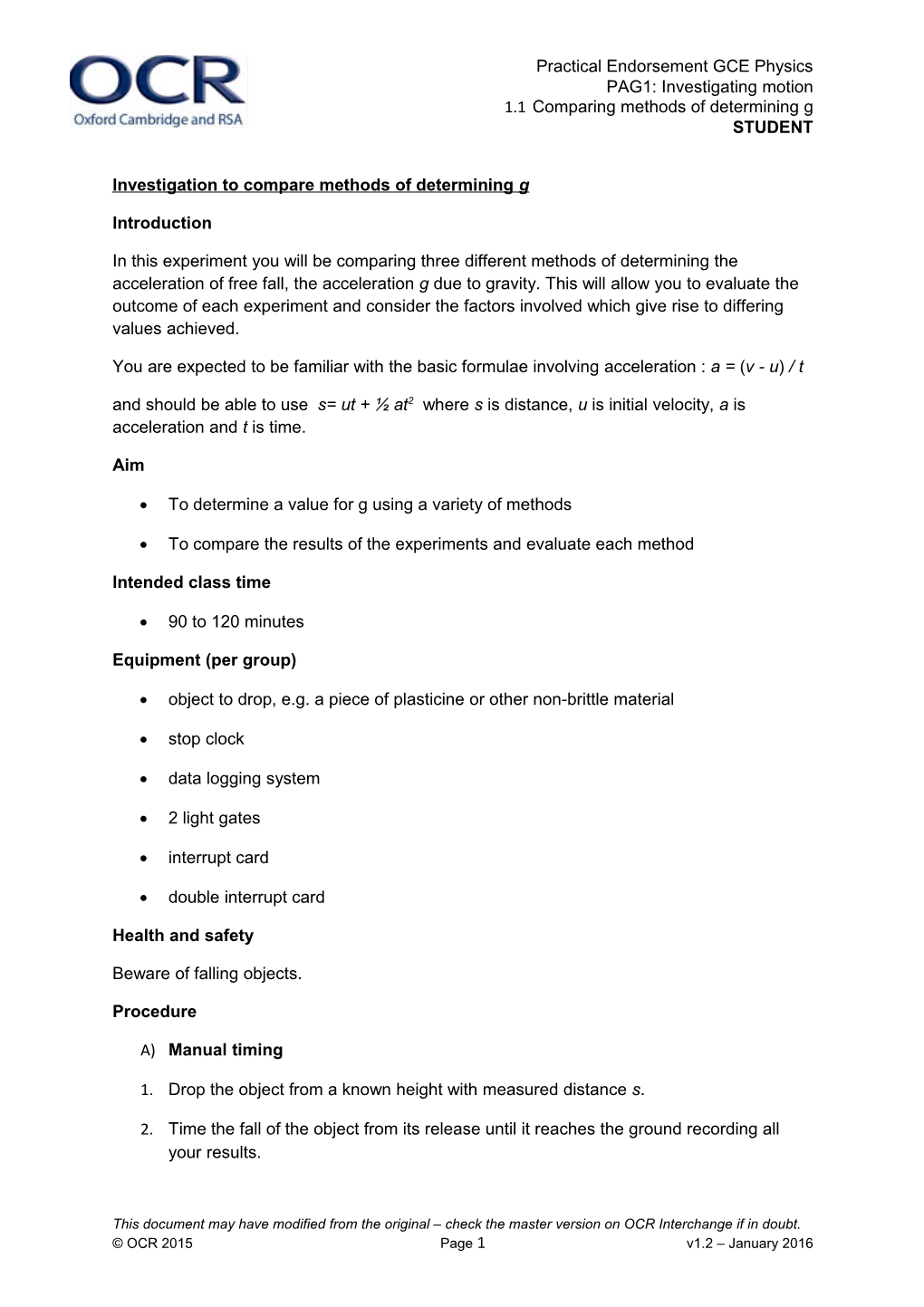# Investigation to Compare Methods of Determining GPractical Endorsement GCE Physics

PAG1: Investigating motion

1.1Comparing methods of determining g

STUDENT

Investigation to compare methods of determining g

Introduction

In this experiment you will be comparing three different methods of determining the acceleration of free fall, the acceleration gdue to gravity. This will allow you to evaluate the outcome of each experiment and consider the factors involved which give rise to differing values achieved.

You are expected to be familiar with the basic formulae involving acceleration :a=(v-u)/t

andshould be able to use s= ut + ½ at2 where s is distance, u is initial velocity, a is acceleration and t is time.

Aim

• To determine a value for g using a variety of methods
• To compare the results of the experiments and evaluate each method

Intended class time

• 90 to 120 minutes

Equipment (per group)

• object to drop, e.g. a piece of plasticine or other non-brittle material
• stop clock
• data logging system
• 2 light gates
• interrupt card
• double interrupt card

Health and safety

Beware of falling objects.

Procedure

A)Manual timing

1. Drop the object from a known height with measured distance s.
2. Time the fall of the object from its release until it reaches the ground recording all your results.
3. Repeat the experiment to validate the results.
4. Calculate the acceleration due to gravity.
5. Note how you have dealt with the following

i)designing your experiment to reduce errors

ii)reducing error in how you take each of your measurements

iii)the possible effects of terminal velocity

B)Acceleration from A to B

1. Set up the data logger with two light gates vertically above each other.
2. Test the system to ensure that the interrupt card falls easily between the two sensors without hitting either sensor or falling at an angle other than vertical, a plumbline may be used to ensure vertical alignment.
3. The logger will require the length of the interrupt card to allow it to calculate velocity at both A and B based on the time it takes to pass through each light gate, and thus calculate speed at each point from the expression: length of card/time to pass through.
4. Set the logger running and drop the interrupt card.
5. Repeat by continuing to drop the card. The logger should record a value for each drop. (If the card misses a light gate or the interrupts get out of sequence, passing the card through light gate B only may get the sequence back to the start.)

C)Acceleration at A

1. Remove light gate B, leaving only light gate A.
2. You will need to use a double interrupt card, which is often a larger square with a gap in the centre. Some interrupt “cards” are clear plastic with black strips top and bottom. The logger will only need the value for one “leg”, top and bottom should be equal, of the interrupt card.
3. Set the logger running and record a series of values for g.

Extension Opportunities - Evaluating the Outcome

1. Make a simple observation of the values obtained by each method.
2. Which method gave the most accurate result compared to the actual value of 9.81 ms-2? Quantify the percentage discrepancy.
3. Which method gives the most instantaneous measure of speed?
4. Why does the logger not require either the distance between sensors or distance across the double interrupt card? (hint a=(v-u)/t )
5. For B and C does it matter at what velocity the card enters the first light gate?

Recording

As evidence for the Practical Endorsement you should have the data collected from your group in a clear and logical format. All work should be clearly dated.

In addition, in preparation for the assessment of practical work in the written examinations and to help develop your understanding of physics, you should have used the data collected to calculate a value for g, explaining clearly how you have used the data in each calculation.

You should have calculated the percentage difference between your calculated values and the accepted value.

You should be able to identify sources of uncertainty in each method and link these to an evaluation of each method.

This document may have modified from the original – check the master version on OCR Interchange if in doubt.

© OCR 2015Page 1v1.2 – January 2016